﻿ Electron's anomalous magnetic moment two-loops correction by QED

# QED anomalous magnetic moment ( 2-loop ) is "fake".

## Quantum electrodynamics (QED) gives "infinity". ← unreal.

### [ Bare "charge" and "mass" of an electron are infinite !   ← unrealistic QED. ]

(Fig.1)   Tiny g-factor is gotten by "artificially" removing "infinity" (= renormalization ) !?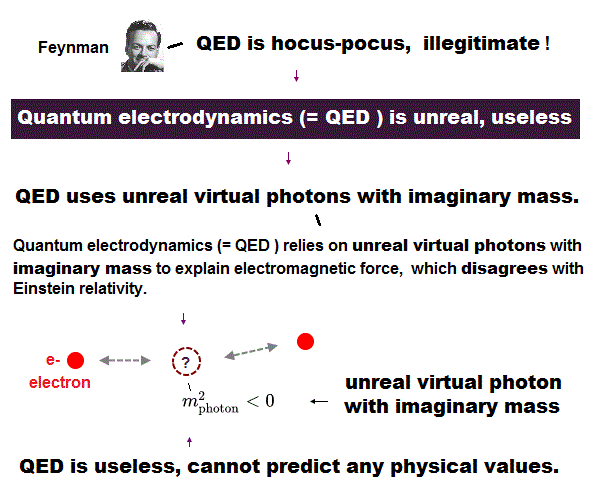Though it is said that quantum electrodynamics (= QED ) is the most successful theory in predicting anomalous magnetic moment (= g-factor ) and Lamb shift, these explanations are NOT true.

In QED, they just artificially remove infinity to leave only convenient finite terms by renormalization.
Because they argue that infinite bare charge and mass of an electron conveniently cancel infinity to generate finite physical values.

In fact, Paul Dirac said, "I am dissatisfied with the situation, because QED neglects infinities in an abitrary way, it is NOT sensible mathematics".   Feynman also called this QED "hocus-pocus".

The magnetic moment of an electron spin is equal to Bohr magneton (= μB ).
They defined spin angular momentum as 1/2 ħ instead of classical "1 ħ", so g-factor becomes twice ( 12 ).

The magnetic moments (= g-factor × angular momentum ) are the same in Bohr's orbit and electron's spin.
Because, magnetic moment = 1 ħ × 1 (= Bohr's orbit g-factor ) = 1/2 ħ × 2 (= spin g-factor ).

It is natural that there is some small difference between ideal g-factor 1 ( or 2 ) and the actual value.
They argue this tiny difference (= 0.001596 .. ) can be explained by unrealistic QED, which always gives infinity.

## QED "abuses" infinity to get convenient "finite" values.

### [ "Splitting" the infinity into "unneeded" infinity and "needed" g-factor. ← Trick ! ]

(Fig.2) Artificial trick of QED = leaving only "convenient" finite values.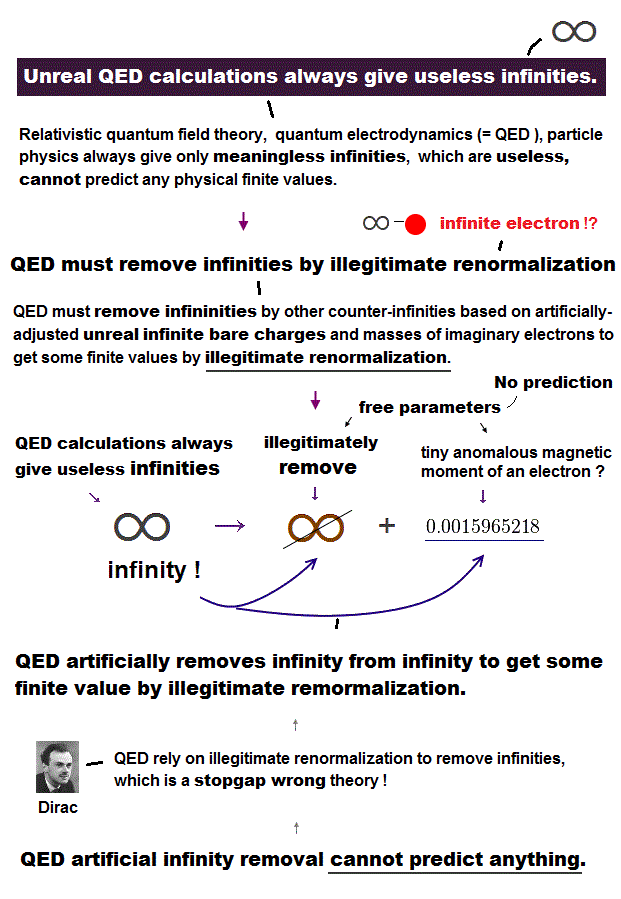When QED calculation contains "loop" in Feynman digram, it always become divergent to infinity.
Of cource, we have to remove these divergence somehow to get physical finite values such as magnetic moment.

So physicists justified the unreasonable equation of " infinity = infinity + finite value ", and have applied this math trick to various important values since 1940s.

As you ( and Dirac ) noticed, these are only artificial and "stopgap" measures.
Because "infinity" can absorb ( or eject ) any "finite" values, so they abuse this logic to obtain convenient finite values.

## One-loop correction of g-factor used math "trick".

(Fig.3) Renomalization of one-loop Feynman diagram.In QED Feynman diagram, they connect electron-electron and photon-photon at each vertex consisting of 1 photon (= ends of dotted line ) and 2 fermions (= ends of solid line ).   The internal lines show propagators and virtual particles violating special relativity.

In 1947, Schwinger obtained α/2π ( α = fine structure constant ), which is close to actual g - 2 factor. Because this Schwinger's g-factor is the first prediction by QED, they could establish new rules to get this value.

So this g-factor correction is NOT natural result of QED, but an artificial result thanks to new rules such as propagators, "gauge" and renormalization.

Of course, using only these new rules, they could NOT obtain good result, so they had to rely on the wrong math trick (= artificial change of variables ) to be close to the experimental value.

## Two-loop correction was much more artificial and doubtful.

### [ Why they couldn't find the first miscalculation (← ? ) for as many as 8 years ? ]

(Fig.4) 2-loop correction summing 7 Feynman diagrams was a mistake in calculation ?An year after Schwinger's one-loop g-factor, Karplus and Kroll calculated its two-loop corrections consisting of 7 Feynman diagrams in 1949.
According to this site, their results were wrong, and Petermann corrected its error eight years later ( in 1957 ).

But as you think, it is very unnatural that the physicists all over the world left the error as it was, for the whole 8 years !   Because just 7 Feynman diagrams do NOT need as many as 8 years for its calculation.

Because, even if you calculate two-loop Feynman diagrams in the same way as the one-loop corretion gotten by Schwinger, you cannot obtain the corret results.

They needed to invent new other artificial rules to get satisfying results.
8 years was necessary for developing the new methods, NOT only for its calculation.

## Artificial "change of variables" changes the calculation result !

### [ Change of variable in "infinite" values (= k ) gives the same "infinity" (= l ).]

(Fig.5) "k" is from -∞ to +∞ → Change of variable → "l" is also from -∞ to +∞.One of the most artificial tricks in one loop correction is "change of variable" from "k" to "l".
Here the part of "yq - zp" is finite variable, and the original virtual relativistic momentum "k" is from -infinity to +infinity.

These propagators including "infinity" values in momentums give ultraviolet divergence. See this and this (p.2).   They replaced the variable "k" with "l", their difference is finite (="yq-zp" ).

The virtual momentum "k" is from -∞ to +∞.
So the resultant "l" is also from -∞ to +∞, because the finite value is absorbed into infinity, and gone.

## "Change of variable" does NOT change the result.

(Fig.6) The integral with respect to "k" ( or "l" ) becomes "zero".In Fig.6, the denominator becomes "even" function, because ±∞ remain ±∞ even after "change of variable" ( k → l ).   And the numerator is "odd" function with respect to "k" ( and "l" )

As a result, this integral from -∞ to +∞ becomes ZERO, irrespective of "change of variable".
This is a right math. You can confirm this, performing integration by parts.

## Trick = "change of variable" changes the result !

(Fig.7) Change of variable ( k → l ) "changes" the result !   ( zero → NOT zero ).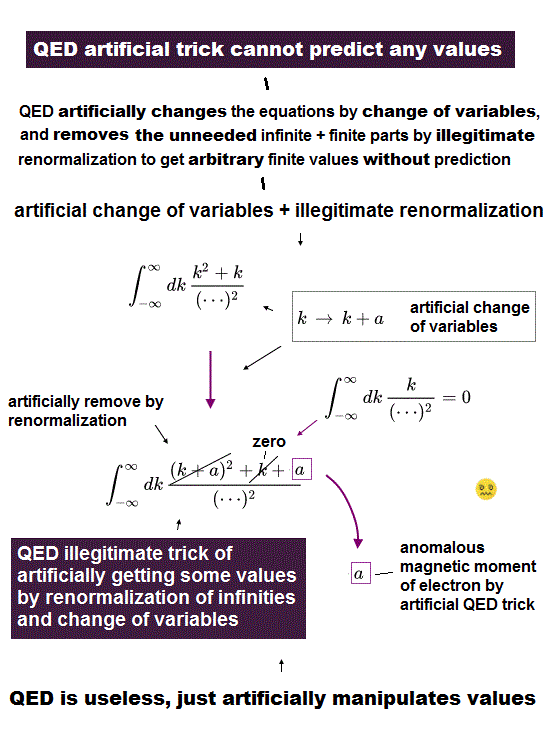But in QED calculation which Schwinger performed, wrong mathematics is used.
After the change of variable ( k → l ), only the denominator used right math.

In numerator. they consider only "l" included in k = "l" - yq + zq as odd function.
As a result, in this wrong math, the finite value (= -yq+zp ) is left, which gives a part of g-factor, they insist.

Originally, the numerator "k" is genuine odd function from -∞ to +∞
So the remaining finite value (= -yq + zp ) in the numerator is very artificial trick, and just wrong.

About the actual calculation, see this (= Eq.7, 14, 15 ) this (p.98) and this.

## QED is mathematical trick.

### [ QED just "intentionally" tries to make calculated values agree with experiment. ]

(Fig.8) Mathematical trick = Change of variable affects the calculated results !?As shown in Fig.8 simple example, "change of variables" ( k = l - β ) artificially changes the result itself, though it's just calculation method.

This is completely mistake in calculation. Of course, "intentional" mistake.
Lamb shift also uses this g-factor value as its part, so QED Lamb shift is wrong, too.

And they try to add various artificial effects to fit them with experimental results ( ex. virtual excited energies in Lamb shift nonrelativistic part ), which means this result is NOT natural.

Of course, if special relativity and Dirac's hydrogen are wrong, QED Lamb shift must be replaced by other interpretations.

## Two-loop correction adopted "new" artificial rules.

### [ 1-loop = only vertex, 2-loops = vertex + mass + propagator renormalization ! ]

(Fig.9) New rules = "mass" and "propagator" renormalization are artificial trick in 2-loop correction.In one-loop correction of anomalous magnetic moment, they could obtain value of α/2π, counting on wrong math.
And this one-loop correction is the first QED calculation, so we can say this whole method itself is new rule.

In two-loop correction of g-factor, they had to rely on other new concepts such as "mass" and "propagator" renormalization.
Depending on new rules means this 2-loop calculation is also artificial, and NOT first-principle at all.

Click "L7-KarpusKrollPhysRev.77" button, then you can get this paper (= Karplus and Kroll ).

The mass and propagator-renormalized terms in this Karplus paper are the same as latest Petermann's paper ( in IId and IIe two-loop diagrams ).

## Redefinition of new propagators by removing "unneeded" finite values.

### [ Removing not only "infinite" terms but also a part of "finite" term ! ← Trick ! ]

(Fig.10) Separating "finite" values into "unneeded" and "needed" parts ← artificial trick.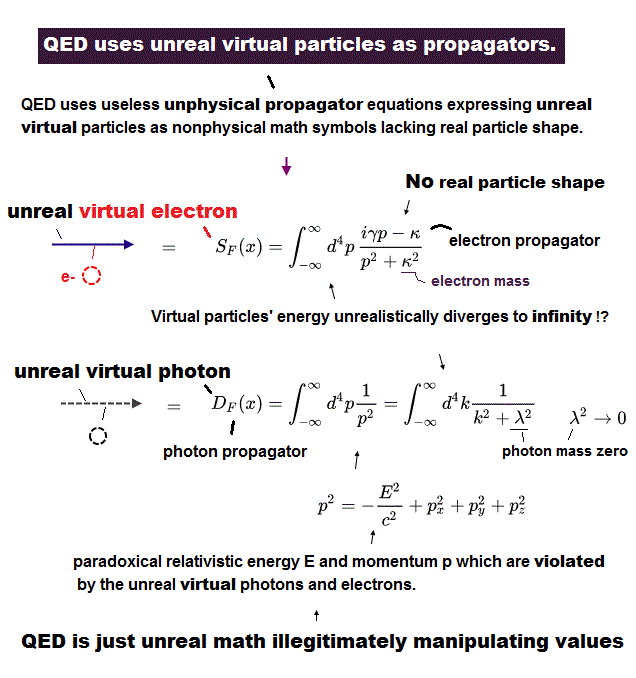The most important tricks in 2-loop correction is the redefinition of propagator ( of photon and electron ).
As shown in this paper (= Eq.11, 12, 13 ), they replaced the original photon propagator (= DF ) with new artificial one by removing unnecessary terms.

The problem is that they eliminated not only infinity but also a part of finite values to obtain g-factor.
There is NO fixed rule about this elimination, so they could use almost "free" ways, as they liked.

And of course, this new method is NOT seen in the original 1-loop correction.
So it is clearly an artificial math trick to get correct g-factor value also in 2-loop correction.

## New electron propagator (= bar-SF ) is also one of "artificial" tricks.

(Fig.11) Redefinition of electron propagator (= SF ) is one of artificial tricks.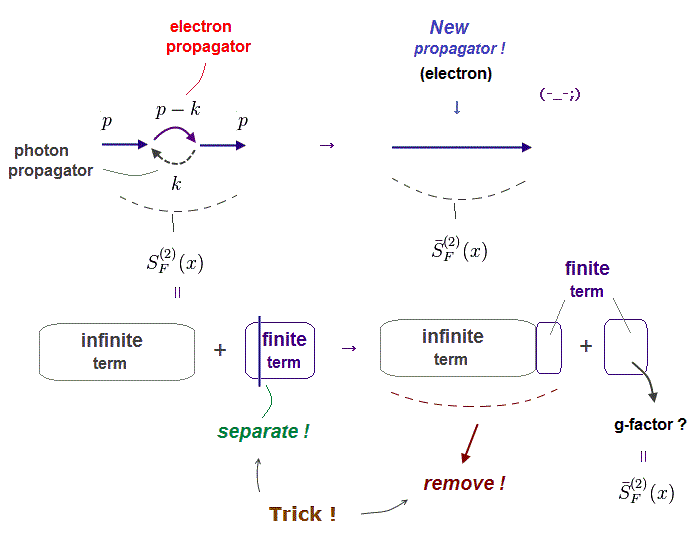As shown in this paper (= Eq.15-23), they replaced electron's propagator (= SF ) with new one.
In this process, they removed not only infinity but also unneeded "finite" values.

Again this renormalization (← ? ) in propagators is an artificial trick.
So this anomalous magnetic moment is NOT first-principle, and does NOT prove the validity of QED at all.

## "Abusing" renormalization in photon self-energy (= propagator ).

(Fig.12) Choosing and removing "unneeded" finite values from photon propagator.As shown in the above paper ( Eq.13, Fig.IIe diagram of FIG.1 ), they eliminated not only infinity (= P ) but also finite values in calculating photon self-energy.

Furthermore, they did NOT mention the content of the finite "P0" term.   There is NO fixed rule here.
About the detailed calculation, see this section.

(Fig.13)   Unnecessary "finite" values are removed, too !The way of separating infinity into "infinite" and "finite" terms is completely arbitrary.
So they abused this "renormalization" of infinity (+ finite value ) to obtain convenient "finite" values.

(Fig.13')  Replaced by "artificial" photon propagator (= bar-DF(2) ).As shown in Eq.36e in the above paper, they replaced the 1-loop part of photon self energy (= Fig.12 ) by artificial photon propagator (= bar-DF(2), Fig.13 ).

So it is clear that they intentionally tried to leave only convenient "finite" values in calculating anomalous magnetic moment.

## Standard photon self-energy is "different" from this 2-loop correction.

(Fig.14) The photon propagator in this paper is "different" from standard form.The photon self-energy consists of two photon's propagators (= dotted line, p ) on both sides and two loop electron's propagators (= solid line, k and p-k ).

The standard photon's self-energy forms are seen in this (p.27) and this (p.199).
The form used in this 2-loop g-factor calculation is completely different from the standard form.

So, there are NO fixed rules stipulating what parts are removed by renormalization.
These free rules allow arbitrary choice and artificial adjustment in calculating anomalous magnetic moment

## "Artificial" adjustment is used also in electron self-energy.

(Fig.15) Feynman diagram of electron self energy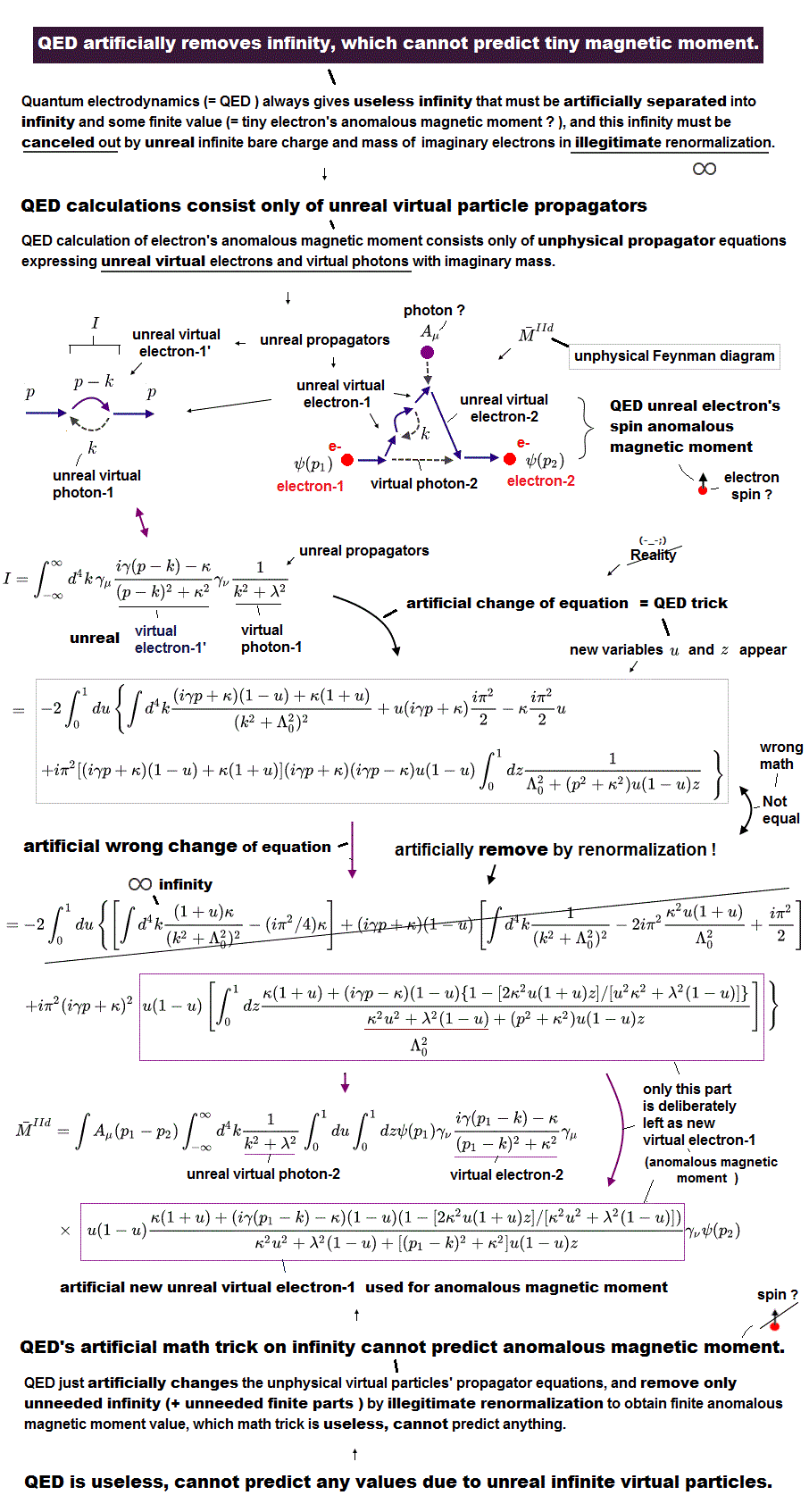The 2-loop correction of g-factor introduced other new concpets such as electron self energy in the above paper ( Eq.15, 16 and IId diagram ).

Feynman diagram of electron self energy consists of two electron propagtors (= p ) on both sides and one loop of a pair of electron (= p-k ) and photon (= k ) propagators.

(Fig.16) ↓ Artificial transformation of equationsIn transforming the equation from Eq.21 to Eq.22 in the above paper, they used non standard calculation method.
As shown in Fig.16, this transformation is unreasonable and NOT correct.

Probably they misused "infinity" for this "unnatural" transformation.

(Fig.17)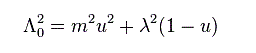Here Λ0 is "finite" value.
"m" and "λ" are electron's and photon's masses, "u" is from 0 to 1.

When this photon mass λ becomes zero, it causes infrared divergence.
Depending on the way of removing this infrared divergence, the calculation result becomes different.

## Artificial removal of unnecessary "finite" terms.

(Fig.18) Not only infinity but also some "finite" term are removed !In Eq.22, 23 in the above paper, they artificially removed not only infinity but also unneeded finite values.

The first term (= "finite" ) of Fig.18 is removed by "mass" renormalization and the second term (= "finite" ) is removed by "propagator" renormalization.

(Fig.19) ↓ Unnecessary "finite" terms are removed !?As shown in Fig.19, they eliminated (= renormalized ) "finite" term, too.

(Fig.20)   Finite value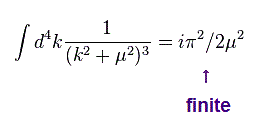As shown in Eq.19 in the paper, the integral of Fig.20 gives "finite" value.

(Fig.20')  Replaced by "artificial" electron propagator (= bar-SF(2) ).As shown in Eq.36d in the above paper, they replaced the 1-loop part of electron self energy (= Fig.15 ) by artificial electron propagator (= bar-SF(2), Fig.19 ).

So again, it is clear that they intentionally tried to leave only convenient "finite" values in using electron self-energy.

## Choice of "mass" or "vertex" renormalizations is "arbitrary".

### [ Which do we use, "mass" or "vertex" renormalizations ? ]

(Fig.21) "Mass" renormalization is a part of vertex renormalization, so meaningless.In 2-loop g-factor correction, they first do mass renormalization to leave only "convenient" finite values.
But as you see, this distinction of "mass" renormalization is meaningless.

Because this mass renormalization (= Fig.21 left ) is only a part of the larger vertex renormalization (= Fig.21 right ).
So distinguishing small "mass" renormalizatin from larger "vertex" renormalization is just an artificial choice.

It is clear that our Nature does NOT depend on these arbitrary and convenient math trick and choice.
So the familiar phrase such as "QED is the most successful theory" is completely wrong, and one of mind-controls.

## Photon self-energy depends on artificial "change of variables".

### [ Trick in calculating photon self energy. ]

(Fig.22)   Photon's self energy is affected by "arbitrary" transformation.In the above paper (Eq.12), they used Schwinger's photon self-energy after a slight modification.
"Modification" means they can change the calculated results as they liked. So it is NOT first-principle.

This Schwinger's paper can be gotten from this site or this ( see appendix, p.25- ).

In this paper (= eq. A.13 in p.25 ), they defined photon-self-energy term (= Gμν ) using two electron propagators (= SF, see Eq.1.66 in p.7 ).

(Fig.23) Two ( virtual ) electron propagators.Fig.23 is ( virtual ) electron's propagators.
k' and k'' are each electron's four - momentum.   We use "m" instead of "κ" as electron's mass.

(Fig.24) "p" = photon's momentum on both sides."p" is photon's momentum on both sides (= "in" and "out" ).
So the sum of k' and k'' should be equal to "p".

## Artificial "change of variables", again !

(Fig.25) They utilized "convenient" change of variables.In this paper (= eq. A.28 in p.26 ), they performed artificial "change of variable".
As you know, "change of variable" is just "means", so it cannot change the result itself according to "right" math.

Here "p" means photon's momentum, and "k" is vitual momentum caused by the difference between two electron's momentums.   ( we exchange "p" and "k" in the above paper. )

(Fig.26) Convenient "infinity" can absorb "finite" values.Using Jacobian determinant of muti-integral, you find this "change of variable" doesn't change the coefficient of integral variables.
But of course, the upper (= +∞ ) and lower (= -∞ ) limits of this integral must change, when they are NOT infinity.

This means they abuse "infinity" to get convenient "finite" value like this section.
Because "infinity" can absorb (or eject ) "finite" values freely.

(Fig.27)In this paper (from A.27 to A.29), they transformed the equation using this change of variables.
Furthermore, they performed partial integral with respect to "v" in A.32, and get

(Fig.28) Photon's self-energy ?See Eq. A.32, A.33, A.35 in the above paper.
This result is almost same as two-loop corretion of Fig.12.

## Different "change of variables" give "different" results !

### [ If we make "v" zero in the original "change of variables", the result changes ! ]

(Fig.29) Artificial "change of variables", part II.Instead of using the original change of variables, we use Fig.29, in which v = 0.
Of course, "v" is variable ( -1 < v < 1 ), so "v = 0" does NOT change the form of the integral.

(Fig.30) "Change of variables" affect the calculation results !But if we use the condition of "v=0" in "change of variables", this photon self-energy becomes zero instead of Fig.28 !   So the means such as "change of variables" affect the calculation results !

This means the results of anomalous magnetic moment (= g-factor ) depend on artificial "change of variables", and are NOT natural things at all.

## Artificial manipulation by QED which is useless.

### [ Artificial trick separating arbitrary finite value (= part of anomalous magnetic moment ) from other infinite value (= removed by artifical renormalization ). ]

(Fig.31) Artificially dividing zero into two ± values (= two red lines ).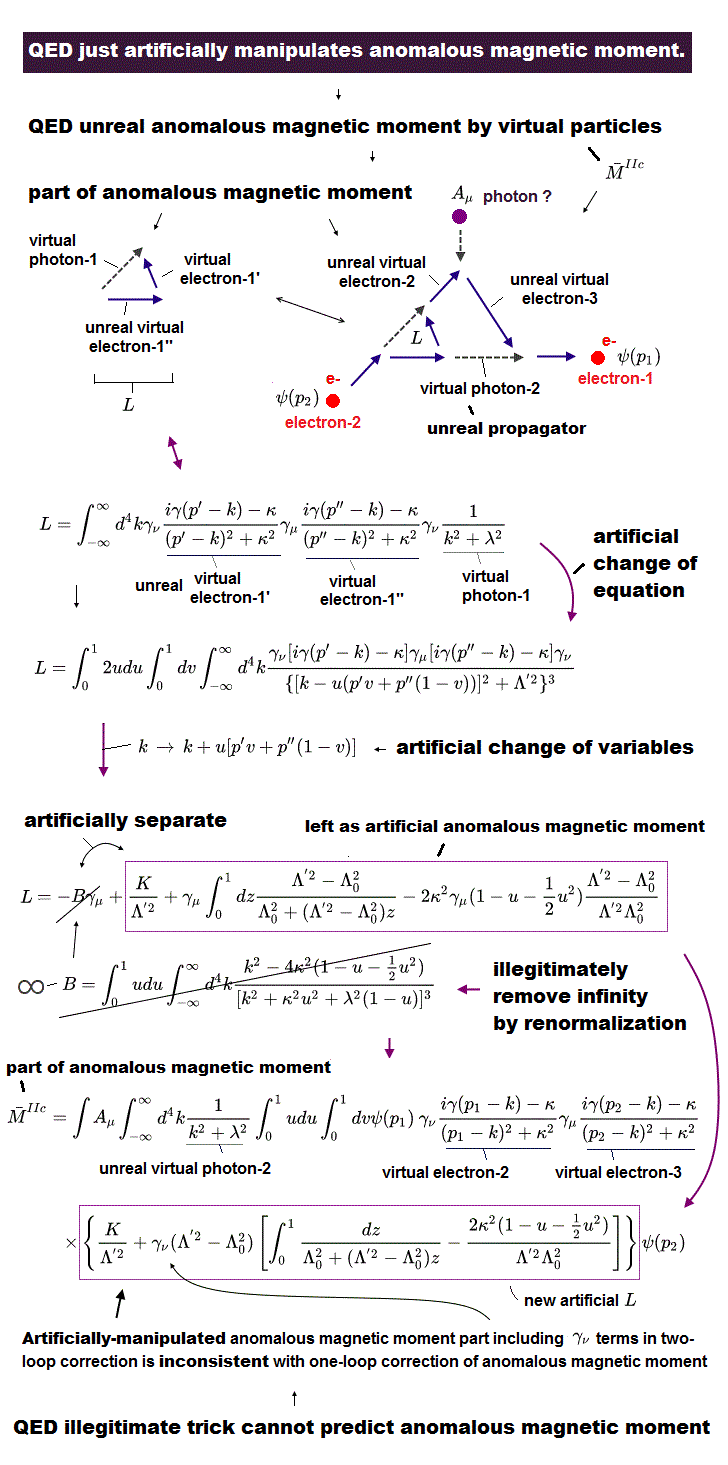Also in some (unphysical) Feynman diagrams of virtual particle's two loop correction ( this p.2 Fig.1 IIa, IIc, and p.7 Fig.5 ~ p.8, and p.9,10 MIIa and MIIc ), QED uses an illegitimate trick artificially manipulating a finite value (= used as a part of anomalous magnetic moment or g-factor of an electron ), which means QED has No ability to predict physical values.

In this p.8 (32) equation, they artificially divide zero into two ±parts (= same value with opposite signs ) and insert only the latter part into the finite part (= become a part of anomalous magnetic moment ).

The former part is artificially removed by being shoved into infinite part B (= removed by renormalization later ), as shown in this p.8 (32), p.9 (33),(36b),(36c).

↑ artificial trick: zero → +a -a  (= only -a part is used as a part of anomalous magnetic moment, and +a part is removed by artificial renormalization ).

This is clearly one of artificial unphysical trick just manipulating electron's anomalous magnetic moment value as they like (= so QED is useless with No ability to predict any physical values ) by diving infinite value into another infinity and arbitrary finite value.

( ↑ In this paper p.8 (32) 2nd equation, the author seemed to forget the coefficient of -γ to the last term. By calculation we can conform equations(28)(30)(32) are the same. This Eq.97 formula is used in (32)-last )2022/1/28 updated. Feel free to link to this site.